﻿ The Effects of Golden Mean on the Diffraction by Monatomic One-Dimensional Quasicrystal

### The Effects of Golden Mean on the Diffraction by Monatomic One-Dimensional Quasicrystal

M. Sa’Id, G. Babaji, S.G. AbduOPEN ACCESSPEER-REVIEWED

## The Effects of Golden Mean on the Diffraction by Monatomic One-Dimensional Quasicrystal

M. Sa’Id1, G. Babaji2, S.G. Abdu3,1Department of Physics, Sa’adatu Rimi College of Education, Kano-Nigeria

2Department of Physics, Bayero University, Kano-Nigeria

### Abstract

In this work, the code ‘Laue’ was used to simulate the diffraction pattern and to investigate the effects of varying the golden mean in a monatomic linear quasicrystal having a pseudo atomic potential. The work involved setting the parameters of the code required to simulate the diffraction, running the code and exporting the data generated to excel for analysis. It was found that the shape of the diffraction pattern and the background intensity for a given value of the golden mean is unique. Both the width of the diffraction pattern and the intensity of the central peak decrease with increasing golden mean. Results obtained illustrated the features of the diffraction by quasicrystal and proved the suitability and accuracy of the code in simulating the dynamics of quasicrystals.

### At a glance: Figures

123
Prev Next

• Sa’Id, M., G. Babaji, and S.G. Abdu. "The Effects of Golden Mean on the Diffraction by Monatomic One-Dimensional Quasicrystal." Applied Mathematics and Physics 2.2 (2014): 53-58.
• Sa’Id, M. , Babaji, G. , & Abdu, S. (2014). The Effects of Golden Mean on the Diffraction by Monatomic One-Dimensional Quasicrystal. Applied Mathematics and Physics, 2(2), 53-58.
• Sa’Id, M., G. Babaji, and S.G. Abdu. "The Effects of Golden Mean on the Diffraction by Monatomic One-Dimensional Quasicrystal." Applied Mathematics and Physics 2, no. 2 (2014): 53-58.

 Import into BibTeX Import into EndNote Import into RefMan Import into RefWorks

### 1. Introduction

The discovery of quasicrystalline phase revolutionized the fundamental concept of crystallography because their diffraction has sharp pattern with rotational symmetry incompatible with periodicity (Shechtman et al, 1984). With this finding, the international union of crystallography has redefined a crystal as any solid material having an essential discrete diffraction diagram. A periodic crystal is formed by a periodic repetition of a single building block, the so-called unit cell exhibiting long range translational orientation symmetry. Only 2-, 3-, 4-, and 6-fold non-trivial rotational symmetries are allowed in the periodic crystals and their diffraction pattern gives sharp Bragg peaks reflecting the symmetry and long range order (Lifshitz, 1997).

In contrast to periodic crystals, quasicrystals exhibit a long range order inspire of their lack of translational symmetry and often possess n-fold (n=5 and >6) rotational symmetries. Most quasicrystalline structures can be described by using quasiperiodic tilling models by employing two or more different unit cells (tiles) used as the building block of the structure (Lifshitz, 1997; Lifshitz and Mandel, 2004). An alternative model of describing quasicrystal is the so-called “covering model” in which a single type but overlapping tiles are arranged to span the entire quasicrystal. These overlapping tiles are related by an irrational number, τ, the so-called golden mean which is related to the geometry of pentagonal and decagonal symmetries. Also in contrast with periodic crystals, three indices are sufficient to characterized the diffraction in 3D structures, n integer indices (n>3) are required to generate the diffraction vectors of a periodic crystal (Lifshitz, 2003).

Fibonacci sequence is a fundamental and a well-known example of a 1D quasiperiodic structure exhibiting a periodic long range order and for whichAlthough it does not feature orientation symmetry, the Fibonacci sequence illustrate many important properties of quasicrystals which can be generalized to 2D and 3D quasicrystals. Fibonacci sequence is built from two elements; b (large) and a (small). The sequence can be generated by a substitution ruleand. The resulting sequence is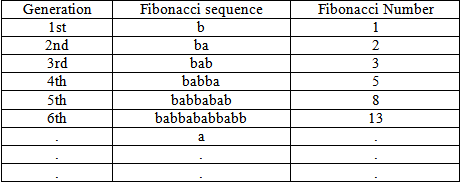The frequencies of b and a in each sequence and the ratio of the successive Fibonacci number is the golden mean, τ (Lifshitz, 2003).

The history of quasicrystal begins with the paper of Shechtman et al in 1984 titled “Metallic Phase with Long-range Orientation Order and No Translation Symmetry”, where the authors demonstrated a clear diffraction pattern with a fivefold symmetry. The pattern was recorded from an Al-Mn alloy which has been rapidly cooled after melting. In 1985, Ishimasa et al reported twelve fold symmetry in Ni-Cr particles. Over the years, hundreds of quasicrystals with various compositions and different symmetries have been discovered. The first quasicrystalline materials were thermodynamically unstable-when heated. The first of many stable quasicrystals were later discovered making it possible to produce large samples for study and opening the door to potential applications (Cahn, 1996).

In 2004, Ferralis et al investigated the diffraction from One- and two dimensional quasicrystalline gratings on one dimensional quasicrystal using two different approaches: laser diffraction and calculated diffraction method using Fibonacci series. The research suggested that, laser diffraction allows an inductive approach for understanding complex aperiodic structure, and can provide educators with an innovative tool for introducing and extending the traditional concept of diffraction.

Theoretical work on the diffraction pattern for an ideal model of quasilattice in which identical atoms are placed at each point of the lattice gave results that are in agreement with experimental observation (Shechtman et al, 198).

To generate a quasicrystal pattern, so called projection methods have been developed (de Bruijin, 1981). Projection methods are mathematical constructs that project sections of hypercubic lattice onto lower dimensional spaces. The first such projection was given by de Bruijn (1981) as reported by Latvin (1988), where he showed that the vertices of the two-dimensional penrose pattern of darts and kites can be generated by projecting hypercubic lattice into a one-dimensional array.

In this work, the effects of varying atomic spacing ratio are investigated on one-dimensional quasicrystal material using the code ‘Laue’ written by Silsbee and Drager (2005). Laue is based on the projection technique, however, unlike traditional projection work, in which the emphasis is entirely on the diffraction pattern, the code can provide additional data that can be used to characterize the quasicrystal. The Laue code has provision for four types of atomic potential shape. Pseudo potential is the atomic shape that describes the position that does not correspond to an actual atom in a molecule but is some sort of average of position of the real atoms. Pseudo atoms are very useful in molecular modeling for energy calculation. A pseudo atom may have the same properties as a real atom (Ishimasa et al, 1985).

### 2. Diffraction Pattern of Quasicrystal

The diffraction patterns of the quasicrystal consist of a set of Bragg peaks that densely fill reciprocal space in an array with quasicrystal symmetry. Consider the case of one-Dimensional quasicrystal with atomic position of the Nth atom given by;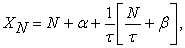(1)

Where τ= golden ratio; α and β are arbitrary real numbers andrepresents the greatest integer function. This particular example is central to studying the pentagonal and icosahedral quasilattice. The atomic position of the 1D (Fibonacci) quasicrystal described by Eqn. (1) may be re-expressed as;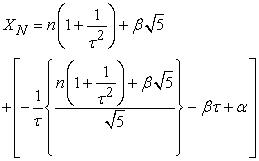(2)

where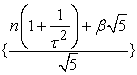signifies the fractional part function and we have used the fact that. An identity isThe function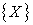is periodic in X with period 1. This expression is of the general form;(3)

whereis periodic in x with period b, and is irrational. Expressions of this variety arise in the study of Frenkel-Kontrova model (Hatano. 1999), which describe a 1D incommensurate crystal. The Fourier transform of such a set of atomic positions consist of Bragg peaks at positions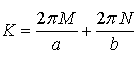(4)

where M and N are integers. This result may be obtained by expanding the exponential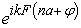appearing in the expression for the transform in a Fourier series of its own and employing the completeness relation for complex exponentials. For our case, this means that there will be peaks at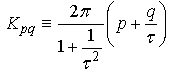(5)

where p and q are integers. With this in mind, we will compute the diffraction pattern (i.e. Fourier transform of the 1D. quasicrystal of Eqn. (2)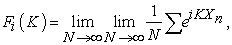(6)

where we are summing over the N atomic position in the chain. First consider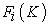for K of the formas define in eqn. (5). Noting the identity, the exponent in eqn. (6) is given by;(7)

The first term in the expressionis an integer timesand therefore only yield a factor of unity upon exponentiation. The last two termsare independent and contribute to the sum in an important fashion. Since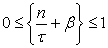, the second termlies between zero and ix, wheresince τ is an irrational number, the value of the second term is uniformly and densely distributed in the interval (0, X), enabling us to approximate the sum in eqn. (6) by an integral;(8)

where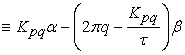and. Equation (8) is the value of Fi(K) for the special values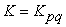, which we argued, correspond to the position of Bragg peaks. Thus we conclude that(9)

Those familiar with the computation of the Fourier transform of 1D quasicrystal via projection method will recognize that the two methods agree exactly. The brightest spots occur for those K=Kpq where X is small. This occurs when q/p is close to τ. It is well known that the best rotational approximants to τ occur when q and p are successive Fibonacci number Fn. This means that the sequence of most intense peaks corresponds to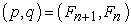(Levine, 1985) .

The diffraction pattern of a quasicrystal is one of its most distinctive features, the pattern consist of a set of Bragg’s peaks that densely fill reciprocal space. This result can be understood intuitively by considering the case of 1D quasicrystal with atomic position given equation (1). The equation can be divided into a sum of two functions that describe periodic spacing but with incommensurate periods. If the first term were kept, the diffraction would consist of Bragg peaks space periodically in reciprocal space with some fundamental period K0. Because the second term is incommensurate, it leads to Bragg peak at some incommensurate reciprocal space period K1. The full pattern then consist of the union of the two sets of peak plus peaks at linear combination of K0 and K1. Because the two are incommensurate, the peaks densely fill reciprocal space in a countable non-fractal way (Levine, 1985).

### 3. Methodology

The code, “Laue” written by Silsbee and Drager (2005) was employed in this work. Basically the code computes the electron density and diffraction pattern of a one-dimensional array of atoms. The one-dimensional crystal is represented by its real-space electron density which is generated from superposition of atomic electron densities. The corresponding diffraction is then computed as the square of the Fourier transform of that electron density. To compute the diffraction pattern (Intensity) of the electron density, “Laue” uses a Fast Fourier Transform (FFT) routine. The intensities are normalized to give a height for the central peak of one for the monatomic quasicrystal (Silsbee and Drager, 2005).

The program has two main output windows for displaying the electron density and diffraction pattern respectively. The code has eight menus namely: Quit, Display, Configure, Presets, Help, Material, Modulation and Calculate. The material menu allows user to select the type of material to be simulated. The possible options are Monatomic crystal, Diatomic crystal, Single atom, Pair of atoms, Liquid and Quasicrystal. In the case of simulating quasicrystal, the program has the following variables: Lattice constant, Size of atom, the Spacing ratio and Atom shape. In this work the atomic potential was first chosen to be Gaussian, and then followed by Pseudo and Exponential and in each, the diffraction pattern was observed and analyzed.

The lattice constant and the size of the atom were set to 4.0 Å and 0.1 Å respectively and the atomic potential was chosen to be pseudo. The atomic spacing ratio was set to be 0.1 and then the simulation executed. The data for the diffraction pattern was then exported to excel for further analysis which includes among others, the computations of the width of the diffraction pattern and the separation of the Bragg planes. This was repeated for atomic spacing ratio of 0.2 to 2.0 Å.

### 4. Results

Figure 1. Diffraction pattern for Quasicrystal using pseudo potential with τ= 0.1 and 0.3

#### Table 1. Variation of width of diffraction patter with atomic spacing ratioDownload asPowerPoint Slide

Veiw figureView current table in a new window

Figure 7. Variation of the Central Peak Diffraction Intensity with Atomic Spacing Ratio

### 5. Discussion

From the Table 1 above it was observed that the width of the diffraction pattern decreases as the golden mean increases. An increase in golden mean decreases the intensity of the central peak as shown in Figure 7. This can be practically predicted that for large number of golden mean the intensity of the central peak will reach zero. However unlike periodic monatomic chain, the diffraction pattern of quasicrystal produces a unique diffraction pattern for each value of golden mean (Figure 1-6). At a value of 1.0 Å for the golden mean (Figure 3), the diffraction pattern resembles the pattern produced by monatomic chain. Also for values of 0.5 (Figure 2), 1.5 (Figure 5) and 2.0 (Figure 6), the diffraction patterns resemble monatomic chain but with growing supper lattices between the already existing peak and hence make the pattern denser than the real monatomic chain. Lastly, it was observed that the density of the diffraction patterns of the above mentioned values are less when compared with golden mean of the remaining values chosen. This is because the supper lattices appeared to have more side bands than the others.

The decrease observed in the intensity of the central peak as the golden mean increases can be attributed to the fact that, the spacing between the ith and (i+1)th atom is given by the lattice constant if the ith position in the Fibonacci chain is 0, otherwise the separation is given by the lattice constant times the spacing ratio. Thus the golden mean (spacing ratio) cannot be infinitely increased because any increase above a certain maximum will result in placing the ith atom outside the ith cell. Thus as the golden mean is increased the separations between the atoms in the neighboring cells decrease, thereby resulting in the decrease in the intensity of the central peak as observed.

### 6. Conclusion

In this work the code “Laue” has been used to simulate the diffraction pattern and investigate the effect of varying the golden mean in a monatomic linear quasicrystal having a pseudo atomic potential. It has been found that:

The shape of the diffraction patterns and the background intensity vary in a complicated manner with the golden mean and that each golden mean results in unique diffraction pattern and background intensity.

The width of the diffraction pattern decreases on increasing the golden mean.

The intensity of the central peak decreases on increasing the golden mean

As expected, the diffraction pattern at the golden mean of 1.0 Å is exactly the same as that of a monatomic one-dimensional crystal.

In conclusion, the laue code has proved to be suitable and accurate in simulating the diffraction pattern of monatomic quasicrystal chain.

### Reference

  Cahn, J.W. (1996), Epiloque: Proceedings of the International Conference on Quasicrystals, edited by C. Janot and R. Mosseri, World Scientific, Singapore, pp. 807.In article  De Bruijn, N,G, (1981), Ned. Acad. Weten. Proc. Ser. A 43, 39-53.In article  Ferralis, N., A. W. Szmodis and R. D. Diehl (2004), Diffraction from One- and Two-Dimensional Quasicrystalline Gratings, American Association of Physics Teachers, University Park, Pennsylvania, 16802, 1241-1246.In article  Ishimasa, T., H.U. Nissen, and Y. Fukano (1985), New Ordered State Between Crystalline and Amorphous Ni-Cr Particles, Phys. Rev. Lett. 55 (5), 511-513.In article CrossRef  Latvin, S.Y. (1988), Generation and Experimental Measurement of One-Dimensional Quasicrystal Diffraction Pattern, Am. J. phys. 56 (1), 72-75.In article CrossRef  Levine, D. (1985), Quasicrystals, Journal De Physique, vol. 46 (8), 397-402.In article  Lifshitz, R. (1996), The Symmetry of Quasiperiodic Crystal, Physica. A 232, 633-647.In article CrossRef  Lifshitz, R. (1997). Theory of Color Symmetry for Periodic and Quasiperiodic Crystal, Rev. Mod. Phys. 69, 1181.In article CrossRef  Lifshitz, R. (2003), Quasicrystal; A Matter of Definition, Found. Phys. 33 (12) 1703-1711.In article CrossRef  Lifshitz, R. and S. E. Mandel (2004), Symmetry of Magnetically Ordered Quasicrystals, Acta Cryst. A 60, 167.In article CrossRef  Shechtman, D., I. Blech, D. Gratias, and J. Cahn (1984), Phys. Rev. let, 53.In article  Silsbee R.H. and Drager (2005), Simulations for Solid State Physics, Cambridge University Press, London, Chapter Three.In article